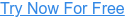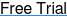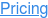#### SOFTWARE#### KNOWLEDGE BASE# What's New in nQuery?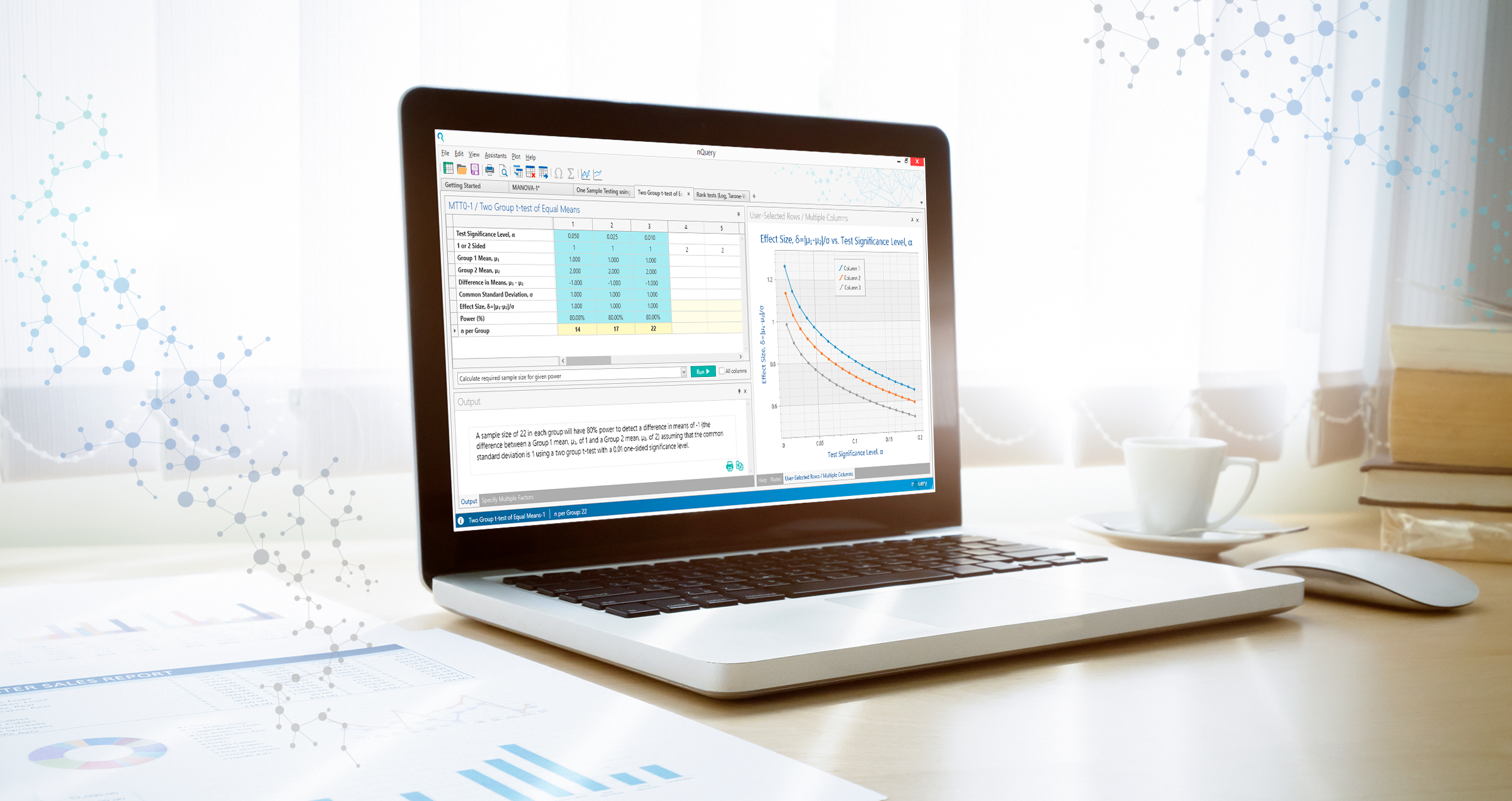The Winter 2021 nQuery release (v9.1) sees
17 new sample size tables and 1 general prediction module update

New Base Tables

#### PLUSTIER

New Bayesian Table

Module update

## The New Prediction Module

(Included in the Expert Tier)

# nQuery Predict

#### Accurately predict your key trial milestones

The Winter 2021 release (v9.1) builds on the latest module for the nQuery platform - nQuery Predict.

These updates focus on UI improvements and further abilities to customize your graphs.

What is nQuery Predict?

nQuery Predict is the latest module for the nQuery platform for clinical trial design.

nQuery Predict uses simulation to help trialists make better predictions about when key trial milestones will occur.

With nQuery Predict, you gain access to tools that accurately predict key enrollment and event milestones both before and while a trial is ongoing. This gives you the ability to identify roadblocks and take action to keep your trial on schedule.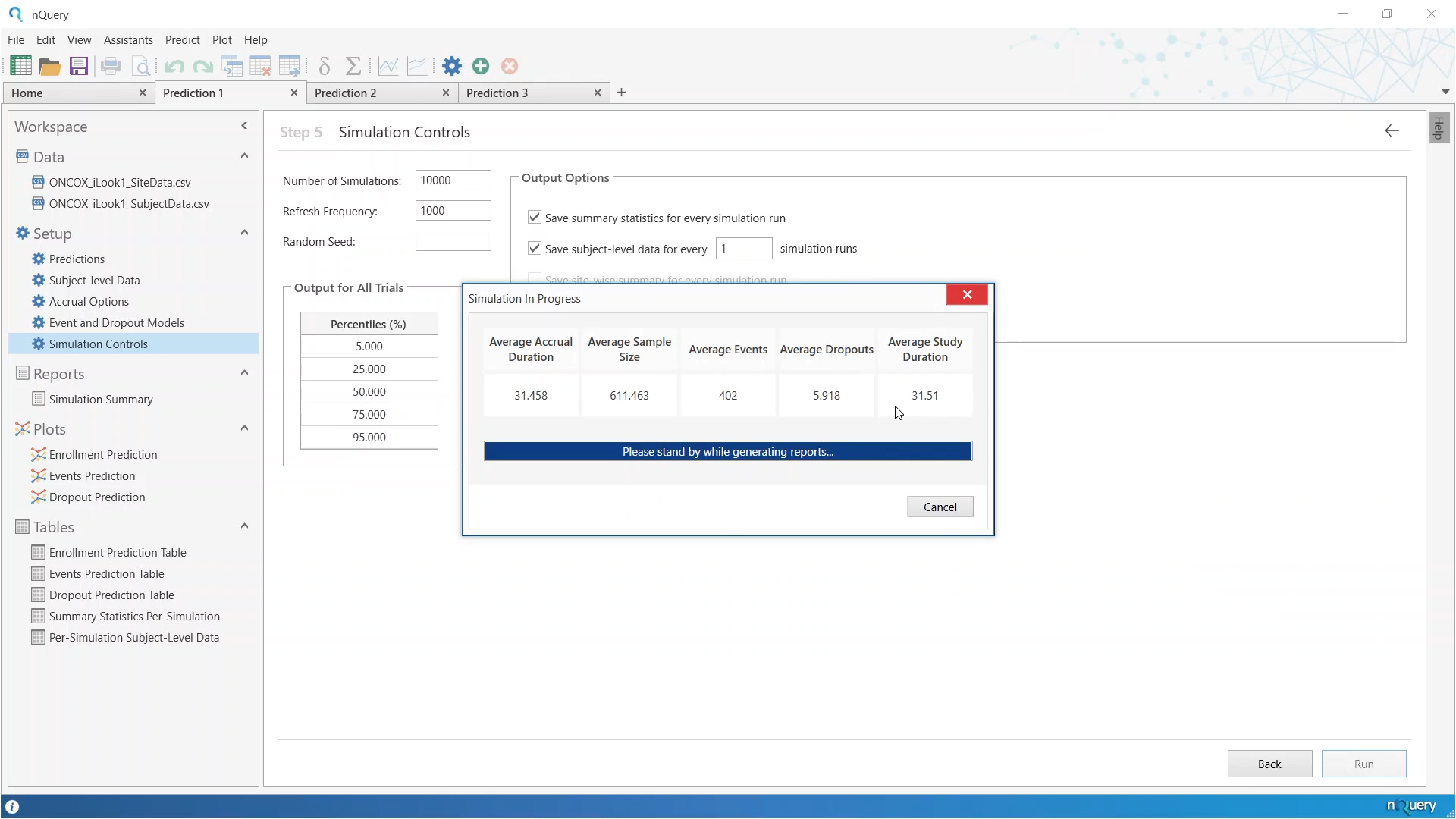### What are the key features of nQuery Predict?

nQuery Predict provides tools that project the expected trajectory of enrollment or event milestones based on current enrollment trends as well as tools to assess the effect of reduced or increased recruitment from that point onwards.

### Enrollment prediction

Enrollment prediction (i.e. how long will it take to achieve the desired sample size of patients) is a common issue across all trials and has direct impacts on the administration of a clinical trial.

Delays in patient enrollment or trial events create additional costs. With nQuery Predict, you can ensure the best performance by examining projections at both the planning stage and interim monitoring.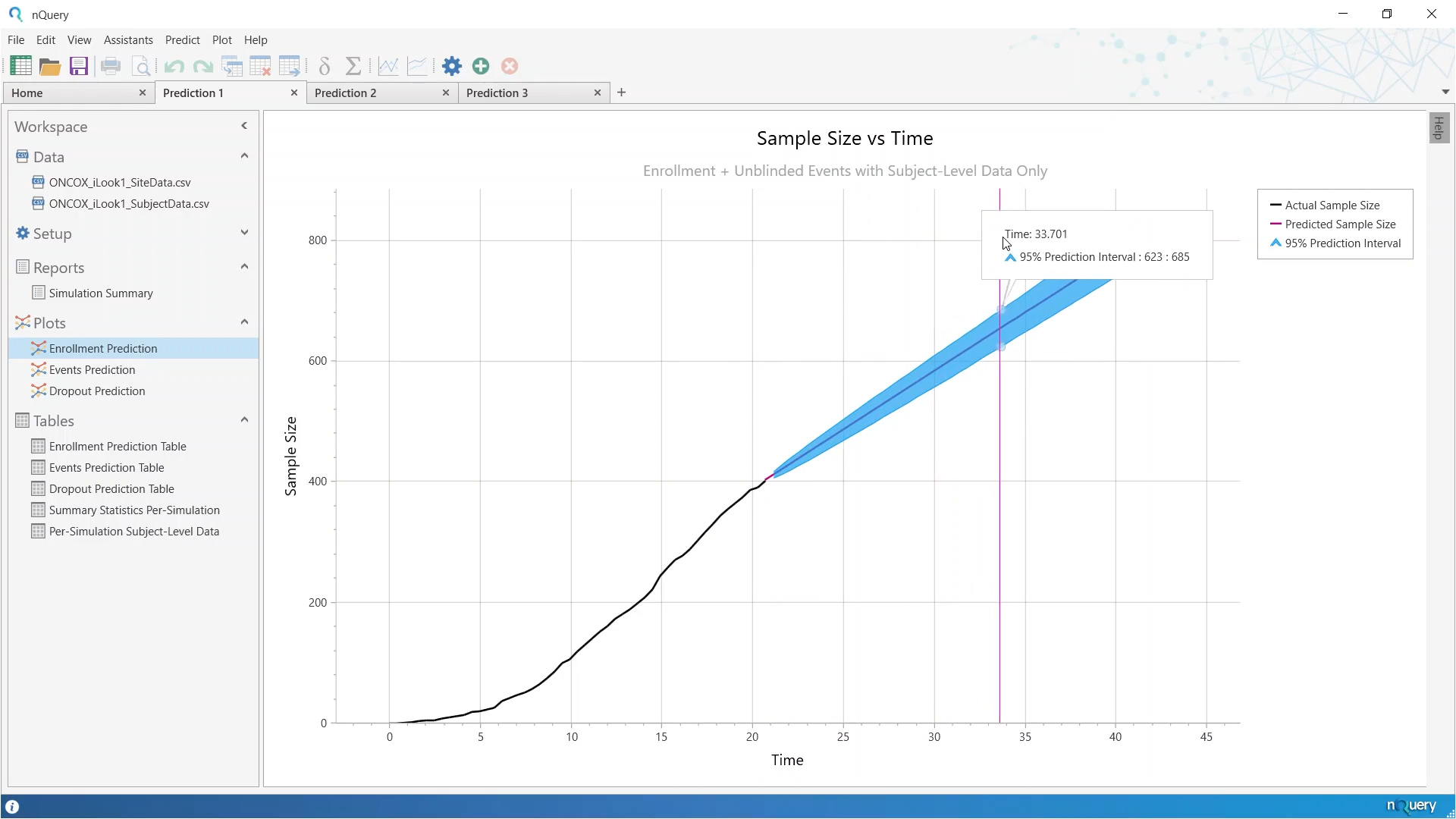### Event prediction

Event prediction is a complex problem in trials, such as those in oncology that plan to use survival analysis.

Key decisions such as when to conduct an interim analysis or when to conduct right-censoring to finish the study are most commonly based on a priori unknown time when a given number of subjects will have had an event rather than based on the fixed follow-up more common in other areas. This means that accurate projections of when event milestones will be achieved are vital to provide realistic estimates of expected timings and costs.

nQuery Predict provides tools to project events based on both blinded (where treatment group is unknown) and unblinded (where treatment group is known) using multiple event models (exponential, piecewise, Weibull) and design considerations (e.g. fixed follow-up per subject).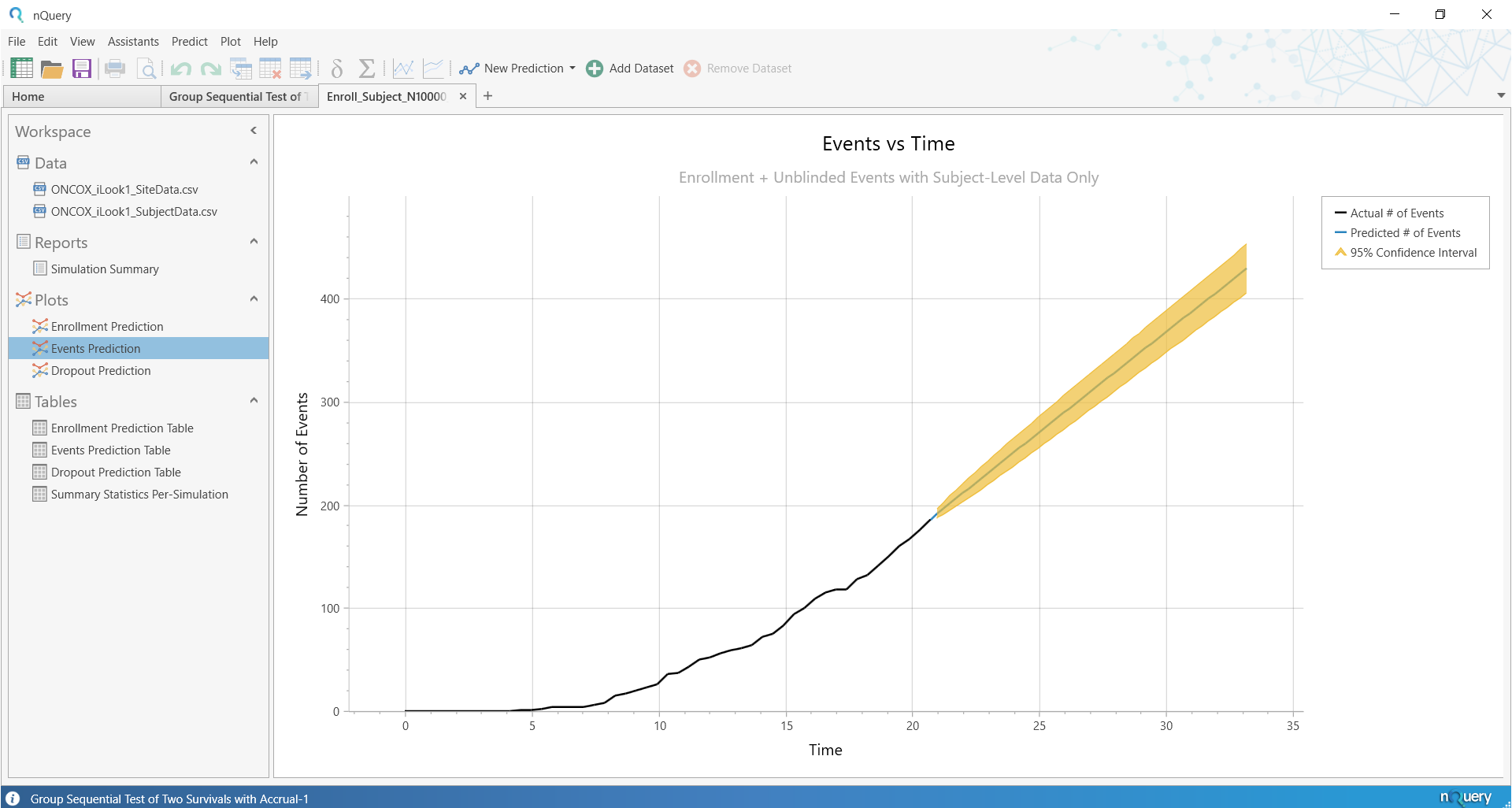### Event & Enrollment Prediction criteria

nQuery Predict uses simulation for milestone prediction with a variety of models for enrollment and events prediction.

##### Event Prediction
• Unblinded
• Blinded
• Exponential Model
• Piecewise Survival Model
• Weibull Model
• Dropout Modelling
• Fixed Followup
• Enrollment Status - Ongoing/ Complete
##### Enrollment Prediction
• Subject-level
• Site-level
• Poisson
• Summary data

## How To Update

To access the new predictive module you must have a nQuery Expert Tier subscription.

If you do, then nQuery should automatically prompt you to update. You can manually update nQuery by clicking Help>Check for updates.## 16 New Base Tables

(Included in all packages: Base, Plus & Pro Tiers)

## What's new in the BASE tier of nQuery?

In the Winter 2021 update, nQuery v9.1 sees 16 new sample size tables added to the base module.

The key areas targeted for development are as follows:

• Proportions Endpoints (5)
• Survival/Time to Event Endpoints (8)
• Sample Size for Prediction Models (3)

#### Tests for Proportions

Proportions are a common type of data where the most common endpoint of interest is a dichotomous variable. Examples in clinical trials include the proportion of patients who experience a tumour regression. There are a wide variety of designs proposed for binary proportions ranging from exact to maximum likelihood to normal approximations.

Under this broad area of proportions, we have added tables across the areas of inequality, equivalence and non-inferiority testing. The general area for these tables are as follows:

1. Non-inferiority Tests
2. Test for Multiple Proportions in One-Way Designs
3. Confidence Intervals for Correlated Proportions

#### Non-inferiority Tests

Non-Inferiority tests are used to test if a proposed treatment is non-inferior to a pre-existing treatment by a specified amount. This is a common objective in areas such as medical devices and generic drug development. The non-inferiority tables added in this release cover the three general types of proportion endpoints one may be interested in for this area.

The first test relates to the difference between two proportions and contains eight options for computing the test statistic, mainly differing in the formula used for the standard error. The test statistics areas follows: Z-Test (Pooled), Z-Test (Unpooled), Z-Test with Continuity Correction (Pooled), Z-Test with Continuity Correction (Unpooled), T-Test, Miettinen and Nurminen’s Likelihood Score Test, Farrington and Manning’s Likelihood Score Test, and Gart and Nam’s Likelihood Score Test.

The second table relates to the ratio of two proportions. IT contains three options for computing the test statistic: Miettinen and Nurminen’s Likelihood Score Test, Farrington and Manning’s Likelihood Score Test, and Gart and Nam’s Likelihood Score Test. Finally the third table added in this area relates to the odds ratio of proportions and contains two options for computing the test statistic: Miettinen and Nurminen’s Likelihood Score Test, and Farrington and Manning’s Likelihood Score Test.

The tables added are as follows:

• Non-Inferiority Tests for the Difference of Two Proportions
• Non-Inferiority Tests for the Ratio of Two Proportions
• Non-Inferiority Tests for the Odds Ratio of Two Proportions

#### Test for Multiple Proportions in One-Way Designs

One-way designs relate to a design in which there is a single independent variable which is manipulated to observe its influence on a dependent variable. One new table has been added in this area. It facilitates one-way designs for the proportion of successes in two or more groups. The methodology used in this table utilizes a likelihood ratio test that has similar power characteristics to logistic regression.

This table assumes the analysis is done using a likelihood ratio test which extends to the case of having more than two independent groups and tests a null hypothesis of the proportion of subjects in each group experiencing the event of interest being equal. This is analogous to the classical one-way ANOVA design that is used to analyze means.

The table added is as follows:

• Tests for Multiple Proportions in a One-Way Design

#### Confidence Intervals for Correlated Proportions

Correlated (paired) proportions occur when two binary variables are obtained for each subject. This may occur when one binary variable represents a pre-test response and the other represents a post-test response. Since both variables are measured on the same subject, it is assumed that there will be a degree of correlation between them.

The table added in this area for the 9.1 release determines the sample size necessary for achieving a confidence interval of a specified width for the difference between correlated proportions. The difference between the two correlated proportions is perhaps the most direct method of comparison between event probabilities. There are five options available within this table for the confidence interval formula. These are the Wald and the Continuity Corrected Wald formulae, Agresti & Min's Wald+2 formula, Bonett & Price's Adjusted Wald formula and Newcombe's Score formula.

The table added is as follows:

• Confidence Intervals for the Difference Between Two Correlated (Paired) Proportions

#### Survival/Time to Event Trials

Survival or Time-to-Event trials are trials in which the endpoint of interest is the time until a particular event occurs, for example, death or tumour regression. Survival analysis is often encountered in areas such as oncology or cardiology. In contrast to studies with non-survival based endpoints, like continuous or binary endpoints for example, the statistical power of a time-to-event study is determined, not by the number of subjects in the study, but rather by the number of events which are observed and this requires additional flexibility when designing and analyzing such trials.

There are many different types of survival designs and in this release, we have chosen to focus on the following main areas:

#### Maximum Combination Test

Combination Tests represent a unified approach to sample size determination for the unweighted and weighted log-rank tests under Proportional Hazard (PH) and Non-Proportional Hazard (NPH) patterns.

The log-rank test is one of the most widely used tests for the comparison of survival curves. However, a number of alternative linear-rank tests are available. The most common reason to use an alternative test is that the performance of the log-rank test depends on the proportional hazards assumption and may suffer significant power loss if the treatment effect (hazard ratio) is not constant. While the standard log-rank test assigns equal importance to each event, weighted log-rank tests apply a prespecified weight function to each event. However, there are many types of non-proportional hazards (delayed treatment effect, diminishing effect, crossing survival curves) so choosing the most appropriate weighted log-rank test can be difficult if the treatment effect profile is unknown at the design stage.

The maximum combination test can be used to compare multiple test statistics and select the most appropriate linear rank-test based on the data, while controlling the Type I error by adjusting for the multiplicity due to the correlation of test statistics. In this release, one new table is being added in the area of maximum combination tests.

The table added is as follows:

• Maximum Combination (MaxCombo) Linear Rank Tests using Piecewise Survival

#### Tests of Relative Time

The concept of Relative Time, as opposed to proportional hazards or proportional time assumes that improvement in a time-to-event endpoint is not instantaneous upon delivery of the treatment, rather, it increases gradually over time. Thus contrary to the assumption of proportional hazard of exponentially distributed times, the effect size is not defined through a single constant number such as a hazard ratio or constant ratios of any two quantiles of time. In this release, one new table has been added in the area of relative time.

The table added is as follows:

• Two Sample Test for Two Survival Curves using Relative Time

#### Stratified Log-Rank Tests

The issue of stratified effects is very common in survival trials, i.e. where there is a different treatment effect for different groups or strata within the trial. These strata often correspond to a prognostic factor (e.g. age group, the severity of disease, gender).

A common method used to combat this issue is the Stratified log-rank test. The log-rank test is a common method for the analysis of survival or time-to-event data in two independent groups. Under a stratified log-rank test, a stratified sample is allowed and a pooled analysis is conducted over the strata. We have implemented one table in this area.

The table added is as follows:

• Two-Sample Log-Rank Test of Exponential Survival with Stratification

#### Test for Interaction Effects

Interaction tests are used in order to evaluate whether the treatment effect differs between subgroups of patients defined by some baseline characteristics. Understanding differences in treatment effects across patient subgroups will help identify patient groups which respond better or worse to the treatment.

One new table has been added in this area for this release. The table added allows for interaction effects between two strata for two different groups and assumes exponentially distributed survival curves.

The table added is as follows:

• Interaction Test for Exponential Survival in a 2x2 Design

#### Tests for One Exponential Mean

Tests for one exponential mean focus on testing differences in mean lifetimes, where it is assumed that lifetimes follow the exponential distribution. One exponential mean test are often used for reliability acceptance testing, which is also known as reliability demonstration.

The sampling plans used within these tests can vary depending on certain choices.

In this release, 4 different tables have been added covering various combinations of these choices. The first of these uses a fixed-failure sampling plan with a fixed sample size. This means that the results are calculated for plans that have fixed sample sizes and are failure censored, i.e. the study terminates when the minimum number of failures that allow the null hypothesis to be rejected occur.

In addition, we have added a table where the results are calculated for plans which are time censored, i.e. the sample size is observed for a fixed length of time. The test statistic used in this table is the mean observed lifetime. Conversely, we have also added a table where the results are calculated for plans that have fixed study durations and are failure censored, i.e. the study terminates when the minimum number of failures that allow the null hypothesis to be rejected occur.

Fixed study duration is used as the termination criterion, which means that the study duration is fixed and the necessary sample size which results in sufficient failures occurring can be calculated. And then finally a table has been added where the results are calculated for fixed-time sampling plans that use the number of failures as a test statistic. This refers to plans whereby the specified number of items are followed for a fixed length of time and the number of failures that occur is recorded.

The tables added are as follows:

• Tests for One Exponential Mean using Fixed-Failure Sampling Plans with Fixed Sample Size
• Tests for One Exponential Mean using Fixed Time Sampling Plans with Lifetime as Test Statistic
• Tests for One Exponential Mean using Fixed-Failure Sampling Plans with Fixed Study Duration
• Tests for One Exponential Mean using Fixed Time Sampling Plans with Failures as Test Statistic

#### Sample Size for Prediction Models

Prediction models are developed to predict health outcomes in individuals and can potentially inform healthcare decisions and patient management. These models are often used in order to predict an individual's risk of having an undiagnosed disease or condition. Typically they are developed using multivariable regression frameworks which provide an equation to estimate an individual's risk based on their values of multiple predictors (e.g. age, smoking, etc.).

From a sample size calculation perspective, the minimum sample size needed in terms of the number of participants and outcome events for a prediction model is of interest here. Multiple different prediction models exist for different endpoints. Three different tables have been added in this release based on these endpoints.

All three of these tables calculate the minimum sample size required based on certain criteria relating to the prediction model. The first of these tables compute the minimum sample size required for the development of a new multivariable linear prediction model with continuous endpoints. For continuous endpoints, linear regression models are typically used in order to predict an individual's outcome value conditional on values of multiple predictor variables.

The second of these tables calculate the minimum sample size needed in terms of the number of participants and outcome events for a logistic prediction model with binary outcomes. Sample size considerations for prediction models of binary outcomes are typically based on blanket rules-of-thumb such as at least 10 Events per Predictor Parameter (EPP), which is the case in this table. Finally, the third table added calculates the minimum sample size needed in terms of the number of participants and outcome events for a Cox prediction model with survival (time-to-event) outcomes.

The tables added are as follows:

• Compute Minimum Sample Size for Linear Prediction Model with Continuous Endpoints
• Compute Minimum Sample Size for Logistic Prediction Model with Binary Outcomes
• Compute Minimum Sample Size for Cox Prediction Model with Survival Outcomes

## List of new BASE Tables

##### Non-inferiority Tests
• Non-Inferiority Tests for the Difference of Two Proportions
• Non-Inferiority Tests for the Ratio of Two Proportions
• Non-Inferiority Tests for the Odds Ratio of Two Proportions
##### Test for Multiple Proportions in One-Way Designs
• Tests for Multiple Proportions in a One-Way Design

##### Confidence Intervals for Correlated Proportions
• Confidence Intervals for the Difference Between Two Correlated (Paired) Proportions

##### Maximum Combination Test
• Maximum Combination (MaxCombo) Linear Rank Tests using Piecewise Survival

##### Tests of Relative Time
• Two Sample Test for Two Survival Curves using Relative Time

##### Stratified Log-Rank Tests
• Two-Sample Log-Rank Test of Exponential Survival with Stratification

##### Test for Interaction Effects
• Interaction Test for Exponential Survival in a 2x2 Design

##### Tests for One Exponential Mean
• Tests for One Exponential Mean using Fixed-Failure Sampling Plans with Fixed Sample Size
• Tests for One Exponential Mean using Fixed Time Sampling Plans with Lifetime as Test Statistic
• Tests for One Exponential Mean using Fixed-Failure Sampling Plans with Fixed Study Duration
• Tests for One Exponential Mean using Fixed Time Sampling Plans with Failures as Test Statistic

##### Sample Size for Prediction Models
• Compute Minimum Sample Size for Linear Prediction Model with Continuous Endpoints
• Compute Minimum Sample Size for Logistic Prediction Model with Binary Outcomes
• Compute Minimum Sample Size for Cox Prediction Model with Survival Outcomes

## How To Update

If you have nQuery installed, nQuery should automatically prompt you to update.If your nQuery home screen is different, you are using an older version of nQuery.

## 1 New Bayesian Table

(Included in the Plus & Pro Tier)

## What's new in the PLUS tier of nQuery?

In the Winter 2021 update, nQuery v9.1 see one new sample size table added to the Plus module.

The area targeted for development is as follows:

• Highest Posterior Density Credible Intervals

#### Highest Posterior Density Credible Intervals

Highest Posterior Density intervals are credible intervals that give the shortest interval length for any given coverage. One new table has been added in this area for this release. This table calculates the sample size needed for two highest posterior density (HPD) credible intervals for a normal mean to agree to within a prespecified distance. The two intervals arise from the use of two different prior densities, each following a gamma distribution. This method is useful in situations where there are multiple prior opinions about the true proportion and we wish to incorporate an “enthusiastic” and “pessimistic” prior into our calculations.

The table added is as follows:

• Consensus Based Sample Size for One Mean

## List of new Bayesian tables

##### Means - One - Confidence Interval
• Bayesian Consensus-Based Sample Size for One Normal Mean

## How To Update

To access these tables, you must have a nQuery Plus or nQuery Pro Tier subscription.

If you do, nQuery should automatically prompt you to update.